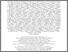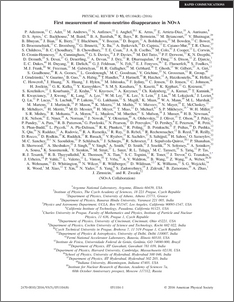# First measurement of muon-neutrino disappearance in NOvA

, NOvA Collaboration (2016) First measurement of muon-neutrino disappearance in NOvA. Physical Review D, 93 (5). ISSN 1550-7998

Other (pdf)
1601.05037v2 - Accepted VersionPreview
PDF (PhysRevD.93.051104)
PhysRevD.93.051104.pdf - Published Version

## Abstract

This paper reports the first measurement using the NOvA detectors of $\nu_\mu$ disappearance in a $\nu_\mu$ beam. The analysis uses a 14 kton-equivalent exposure of $2.74 \times 10^{20}$ protons-on-target from the Fermilab NuMI beam. Assuming the normal neutrino mass hierarchy, we measure $\Delta m^{2}_{32}=(2.52^{+0.20}_{-0.18})\times 10^{-3}$ eV$^{2}$ and $\sin^2\theta_{23}$ in the range 0.38-0.65, both at the 68% confidence level, with two statistically-degenerate best fit points at $\sin^2\theta_{23} =$ 0.43 and 0.60. Results for the inverted mass hierarchy are also presented.

Item Type:
Journal Article
Journal or Publication Title:
Physical Review D